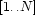Init Method

The init method is given a list of domains. Each domain is represented by the list of its values (these should be atoms or integers). A DomainProduct object is created as follows:

declare O = {New DomainProduct init([D1 D2 ... Dp])}

where each Di is a list of values representing a domain.

The initialization code constructs the map value2set by iterating through the integers in the range. For each I in this range, DecodeInt is invoked to obtain the corresponding tuple (as a list of values). For each value V in this tuple, I is added to the list of indices for V. When we are done, these lists of indices are turned into sets of indices.

meth init(Domains)
Sizes = {Map Domains Length}
L1    = {Map Sizes fun {\$ Size} _#Size end}
N     = {FoldR L1 fun {\$ M#N Accu} M=Accu N*Accu end 1}
Divs  = {Map L1 fun {\$ M#_} M end}
Val2Ints = {Dictionary.new}
in
for I in 1..do
Tuple = {DecodeInt I-1 Divs Domains}
in
for V in Tuple do
{Dictionary.put Val2Ints V
I|{Dictionary.condGet Val2Ints V nil}}
end
end
divisors <- Divs
domains  <- Domains

for K in {Dictionary.keys Val2Ints} do
Val2Ints.K := {FS.value.make Val2Ints.K}
end
self.range = 1#N
self.empty = FS.value.empty
self.full  = {FS.value.make self.range}
value2set <- Val2Ints
end

Denys Duchier
Version 1.2.0 (20010221)